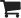The Bar Profit Guys
800-285-2337
\$0.00 (0)MY CART

# Pour Cost Calculators

Find Out if Your Pour Costs are Too High
UNDERSTANDING BEVERAGE COST PERCENTAGES

Bar operators typically evaluate their establishments based on their "cost of goods" percentage � more commonly referred to as �pour cost.� Pour cost is calculated by simply adding up the cost of the product used and dividing it by the cost of the product sold. For example, if a bar depleted \$5,000 of liquor in January and had liquor sales in January of \$25,000, their liquor pour cost for January would be 20% (\$5,000 � \$25,000). A pour cost of 20% indicates that every dollar of sales generated, cost the establishment 20 cents.

The main problem with pour cost is deciding what to compare it against. How do you know if your percentages are in line? Most operators simply look at the average pour costs in the industry or at their previous annual cost percentages. On that basis, most operators are happy if their liquor pour cost is around 15%, draft beer in the neighborhood of 20%, bottled beer close to 25% and wine between 30% - 40%. But even if a bar�s cost percentages come close to these arbitrary targets, they may still be too high.

Managers should compare their ACTUAL cost percentages to their POTENTIAL (or optimal) cost percentages. Potential cost percentages are determined by taking into account an operation's selling prices, purchasing costs, and sales mix.

A. When calculating a potential liquor cost percentage, the desired liquor shot size needs to be considered.

B. When determining potential draft beer cost percentages, the sales mix of each draft beer container used (mugs, glasses, pitchers) has to be figured into the calculation.

C. Determining an operation's potential bottled beer cost percentage is just a matter of dividing a bottle's cost by its selling price. For example, if Budweiser costs an establishment 50� per bottle and is sold for \$2.00 per bottle, then the potential cost percentage is \$.50/\$2.00 = .25 = 25%.

D. Actual cost percentages are the actual costs incurred (taken from distributor�s invoices) divided by the actual sales generated (taken from the cash registers.)

So what does this all mean? If the actual beverage cost percentage is currently at 30%, then gross profit would be 70%. When the actual cost percentage is lowered to 25%, then gross profit increases to 75%. Let's assume a bar operation earns beverage revenues of \$200,000 a year. By improving revenue & cost controls to help reduce the beverage cost percentage from 30% to 20%, gross profits will increase 10%, which is another \$20,000 of additional profits being generated from the same revenue!

CALCULATING YOUR OPERATION'S POTENTIAL COST PERCENTAGES

Below are calculators that will determine your operation�s potential (or optimal) beverage cost percentages. Compare your potential cost percentages to your actual cost percentages to help evaluate your establishment�s lost profits by revenue category.

After clicking on one of the COST PERCENTAGE CALCULATOR links below, an Excel file will open and you will see two tabs at the bottom of the screen. Click on the SAMPLE tab to see an example Cost Percentage Calculation and on the WORKSHEET tab to perform your own Cost Percentage Calculation. If prompted by Excel, click the "Enable Editing" button at the top of the page.

All you have to do is fill in the yellow cells and the worksheet will instantaneously calculate your potential cost percentage. Cells with red flags in the top right corner, provide further explanation when the cursor is placed over the cell.

If you have questions, the Bar Profit Guys can be reached by calling 800-285-2337 between 9am � 5pm EST or emailing Profit@AlcoholControls.com.